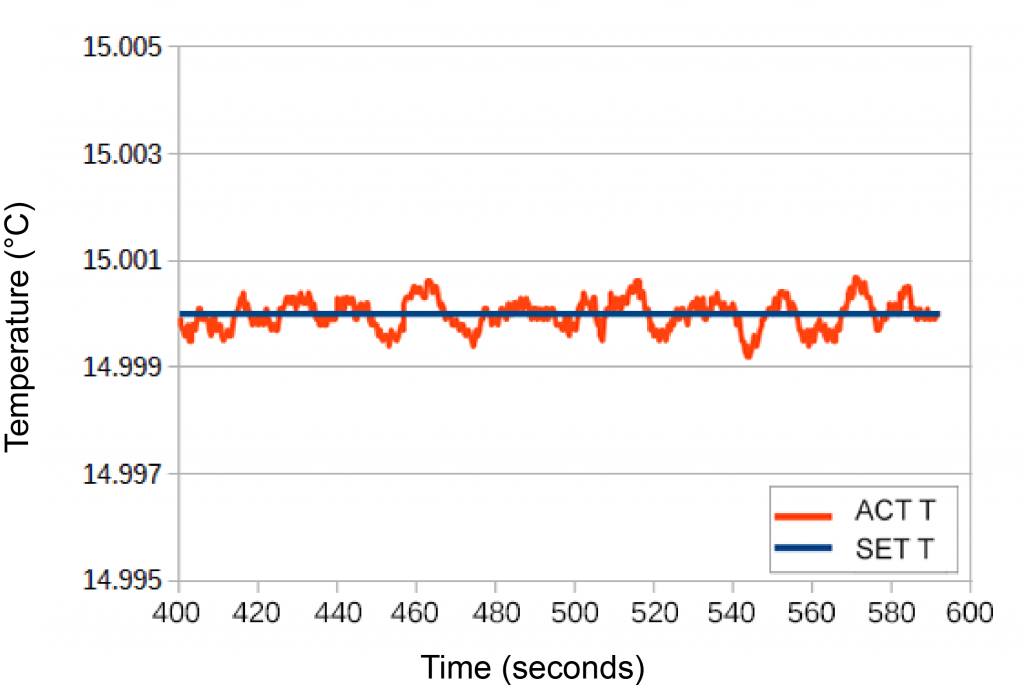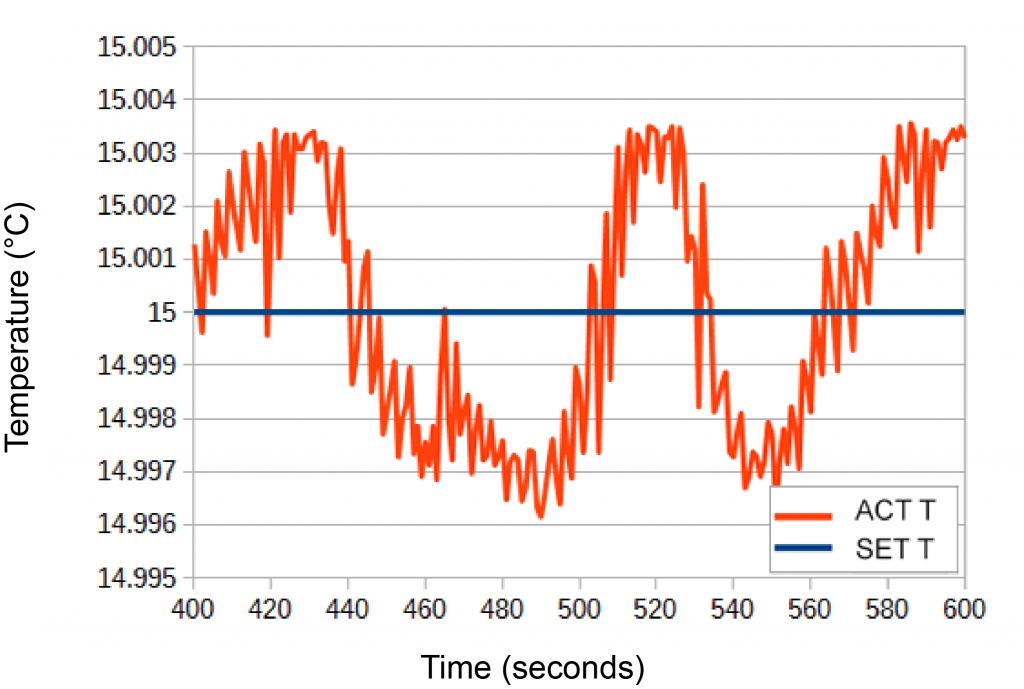TC10 LAB
TC15 LAB

## Question

How does the stability of the TC LAB instrument compare with the competition?

In order to address this question we ran a series of experiments comparing the TC10 LAB using IntelliTune® against three different competitor units using a conventional autotune algorithm.

Each temperature controller instrument used a different autotune algorithm. Most took about two minutes to complete. One took 28 minutes to choose the optimal PID coefficients. Step response varied from underdamped to overdamped. Some algorithms drove the load to the edge of instability to choose PID coefficients. P, I, and D coefficients do not represent the same physical units across instruments.

IntelliTune goes beyond conventional Autotune by recalculating optimal PID coefficients for different setpoints or tuning modes without requiring multiple characterization scans. Control coefficients are chosen for best stability.

Shown below is a comparison of the TC10 LAB (Figure 1) with Competitor B (Figure 2). Competitor C truncated their data resolution at 2 decimal places, so a comparison was not possible.Figure 1. Disturbance Rejection mode Stability was 1.27mK (Three standard deviations over 1500 seconds)Figure 2. Competitor B Compromised Stability Stability was 7.6mK (Three standard deviations over 600 seconds)